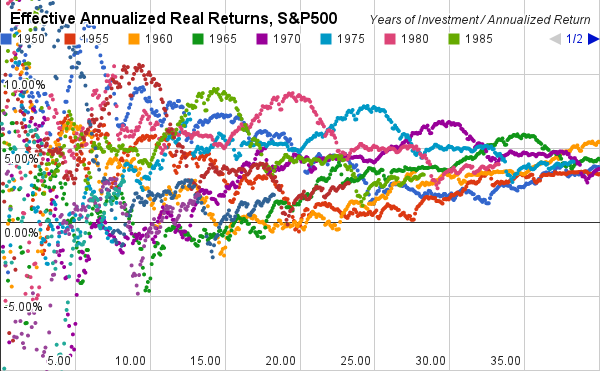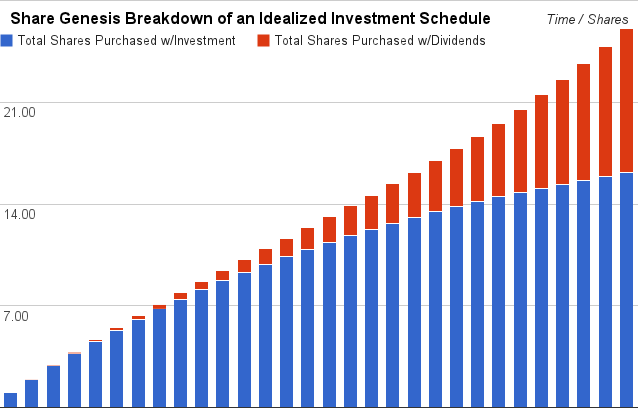## 2013-05-01

### Effective Annualized Return

The Internet is full of people arguing about the stock market.  A question I'm interested in: if my parents or grandparents had invested in an index mutual fund that followed the S&P500 inside a tax-advantaged account such as an IRA or a 401(k), how much money would they have today?

Toward answering this question, I created the SimVestment spreadsheet that I shared in a post last month.  It uses historical S&P500 data to simulate what would have happened if a particular investment schedule had been followed over a period of time in the past.  The spreadsheet takes into account the automatic reinvestment of dividends, adjusts for inflation, and can handle investment rates that change with time; the investment rate can be automatically adjusted to a constant value in 2013 dollars, making the dollar value amounts a lot more meaningful.

As I discussed in the previous post with regards to arithmetic and geometric means, it's very important to clearly define what it is you're calculating. Take, for instance, the graph of annualized return from that previous post. Each color is a different starting year, the x-axis is the number of years invested, and the y-axis is the annualized, inflation-adjusted rate of return:Play with the original graph here
As the graph title states, this visualizes the annualized real return over different time periods.  You could use this information to calculate the net real return of a lump sum invested all at once.

Going into the spreadsheet, I changed the 'initial investment' to zero and set the 'monthly investment' to \$1000, selecting the option to invest at a constant 2013 dollar rate.  The spreadsheet then simulates a situation in which you invest a constant value (in 2013 dollars) every month.  The graph then looks like this:Play with the original graph here

The returns, especially over the shorter investment durations on the left-hand side of the graph, seem to 'tighten up' a bit; similarly, they seem to drop slightly for the longest investment durations on the right-hand side of the graph.

Look carefully at the title in the second graph: the values calculated are Effective Annualized Real Returns.

What does this mean?  The spreadsheet is calculating the effective annual rate of return over the time period as if you had invested all of the money at the beginning.  The effective annualized real return changes with the nature of your investment scheduling over the period, while annualized return only depends on the year you start and the length of the period.

Why calculate this value?  Because this is how people actually invest.

The lump-sum graph tends to give you the wrong idea about the magnitude of the capital appreciation they should expect over a time period when investing an amount of money divided into a number of regular payments.  Yes, the stock market may return ~7% annually after adjusting for inflation, but the effective annualized real return that you see for the total invested over the entire period will be lower because you're investing your money over time.

The difference is better illustrated in an idealized investment model, in which a stock increases 5% in value and issues a 1% dividend every year.  Note the differences in y-axis scale:Lump Sum: visualizes how the invested money, dividends, and capital appreciation factor into the total value over timeRegular Investment: visualizes how the invested money, dividends, and capital appreciation factor into the total value over timeLump Sum: Shows where the owned shares have come from, over timeRegular Investment: Shows where the owned shares have come from, over time

(BONUS QUIZ: Why, at the end of the time period, has the 'Regular Investment' schedule purchased far fewer shares with the invested money (blue bars) than the 'Lump Sum' schedule?)